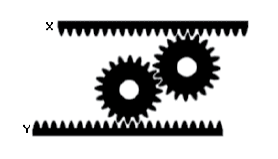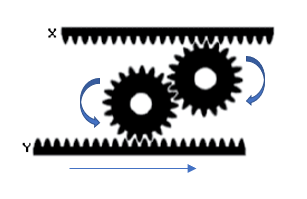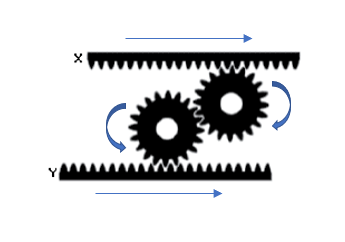## Gear Movement

If the Y bar is moving right at a constant speed, how does the X bar move in terms of speed and direction?HintHint 2
Because the gears are equal size, the ratio is 1:1.Because the gears are equal size, the ratio is 1:1, meaning both the X and Y bars are moving at the same speeds. Visualizing the Y bar moving right, the first gear will rotate counter clock wise, causing the second gear to rotate clock wise. As a result, the X bar will move to the right at the same speed as the Y bar.
To the right at the same speed.